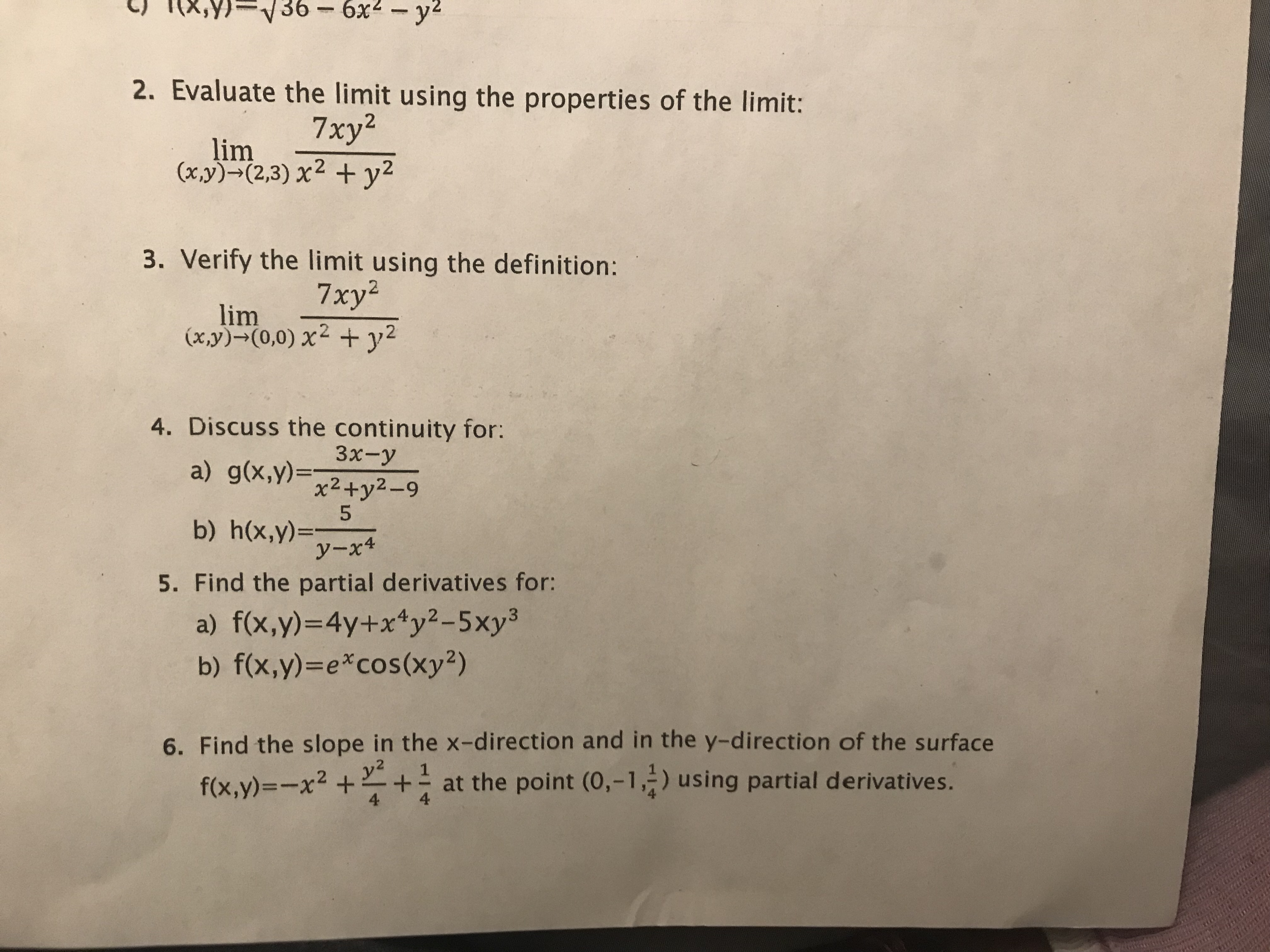# , 36-6x2-y2 2. Evaluate the limit using the properties of the limit: 7xy2 lim (x,y)(2,3) x2 y2 3. Verify the limit using the definition: 7xy2 lim (xy)-(0,0) x + y2 4. Discuss the continuity for: Зх-у a) g(x,y)x2+y2-9 5 b) h(x,y)- y-x4 5. Find the partial derivatives for: a) f(x,y)-4y+xy2 - 5 xy3 b) f(x,y)-e*cos (xy2) 6. Find the slope in the x-direction and in the y-direction of the surface y2 1 f(x,y)-x2++at the point (0,-1,) using partial derivatives. 4 4

Question

2,3,4help_outlineImage Transcriptionclose, 36-6x2-y2 2. Evaluate the limit using the properties of the limit: 7xy2 lim (x,y)(2,3) x2 y2 3. Verify the limit using the definition: 7xy2 lim (xy)-(0,0) x + y2 4. Discuss the continuity for: Зх-у a) g(x,y)x2+y2-9 5 b) h(x,y)- y-x4 5. Find the partial derivatives for: a) f(x,y)-4y+xy2 - 5 xy3 b) f(x,y)-e*cos (xy2) 6. Find the slope in the x-direction and in the y-direction of the surface y2 1 f(x,y)-x2++at the point (0,-1,) using partial derivatives. 4 4 fullscreen

### Want to see the step-by-step answer?